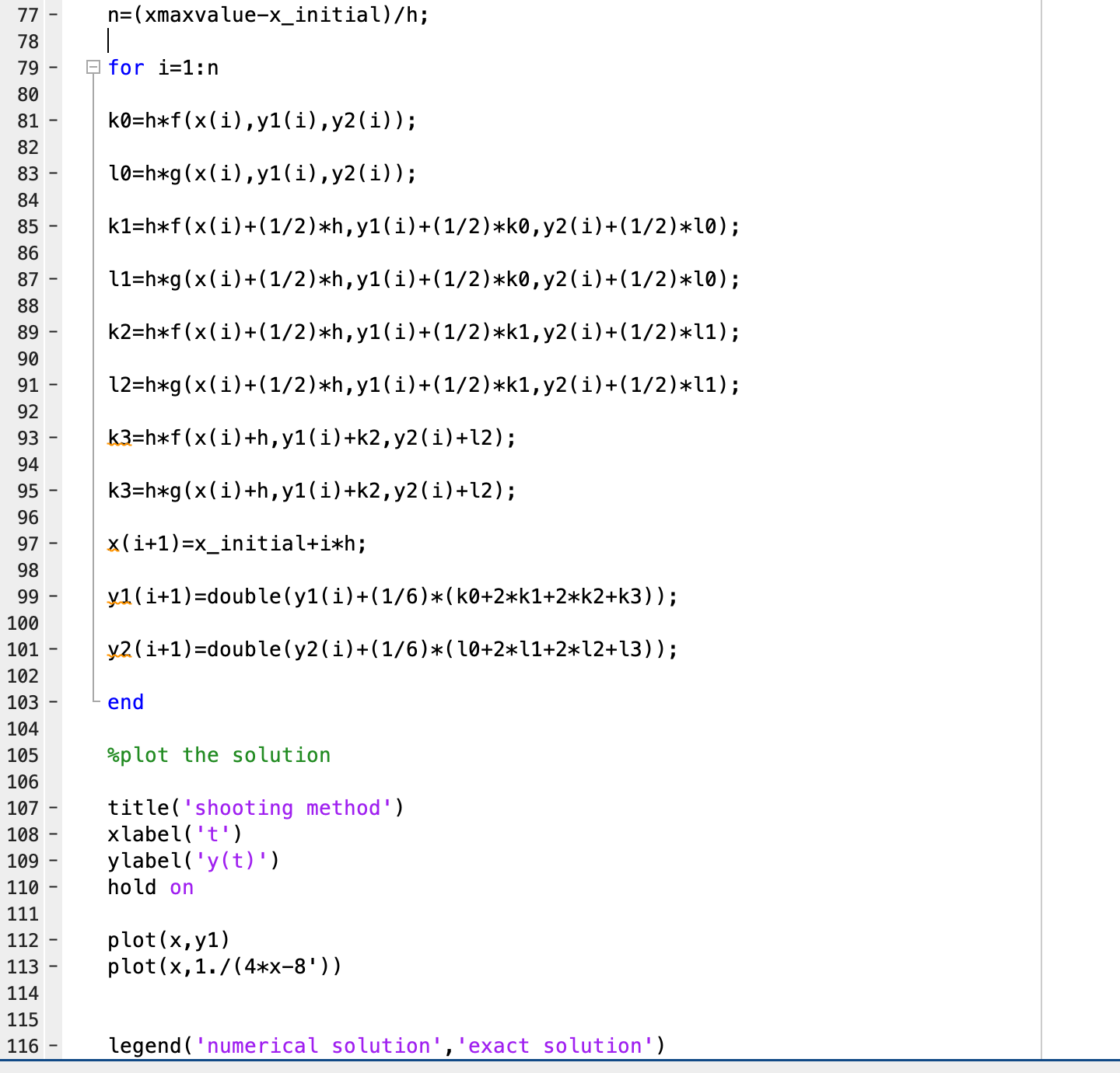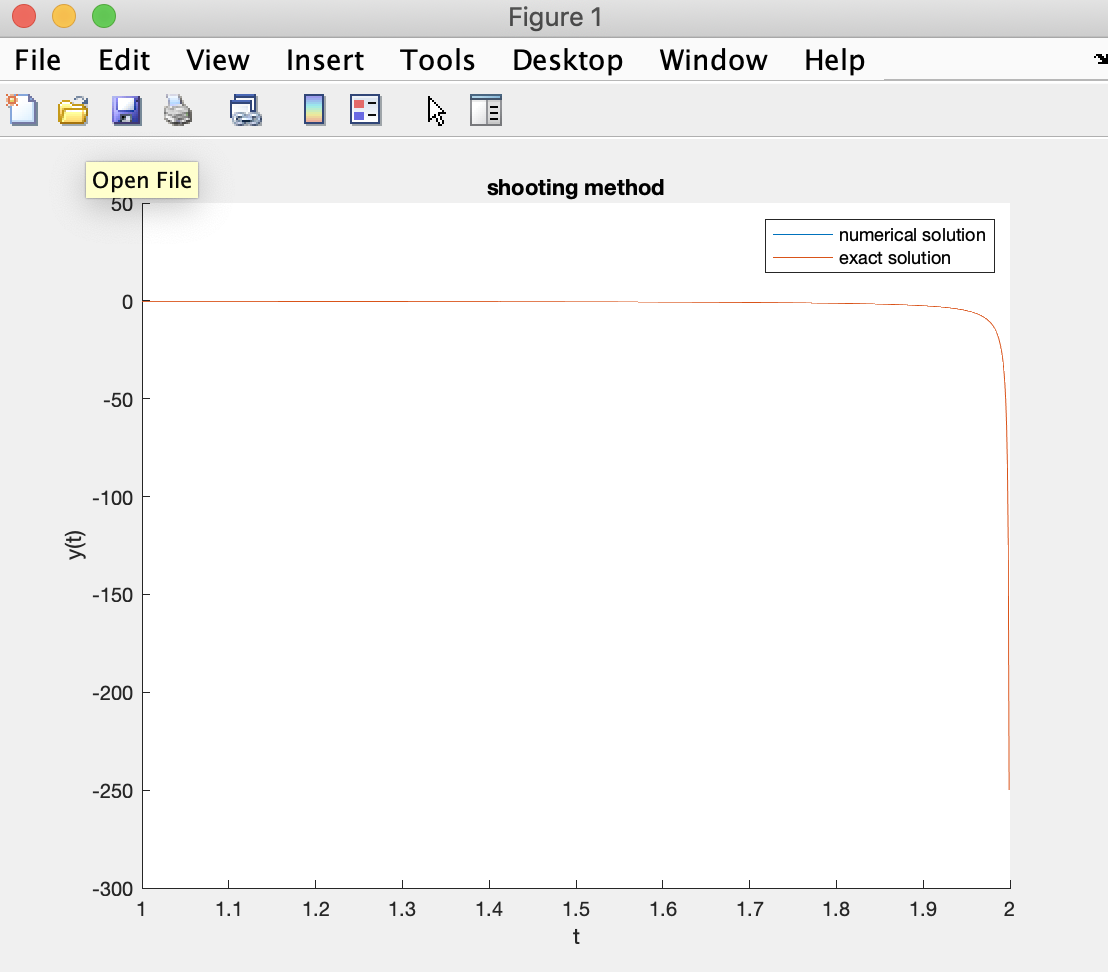# MATLAB plot not showing.

294 views (last 30 days)
jlkoi1234 on 10 Apr 2019
Answered: VBBV on 18 Oct 2021
I tried plotting two graphs with the following:with the following output:The second one (plot(x,1./(4*x-8'))) is showing just fine, but not the first one (plot x,y1). Y1 is a 1x1001 double array. Why is that, and how can i fix the problem?
Rena Berman on 13 May 2019

Jon on 10 Apr 2019
The problem is that the second plot is replacing (overwriting) the first one in the figure window. If you open up a new figure, before making the second plot you will avoid this problem. Even better, create a new figure for each plot in case you already had another one there you didn't want to overwrite, So for example:
% make new figure
figure
% make the first plot
(plot x,y1)
% make another figure
figure
(plot(x,1./(4*x-8')))
Also a couple of other side comments. I agree with Stephen's comment, it is better to use the code button to insert code. Also in your specific plot command, I'm not sure what you are intending with 4*x - 8'. In this case the transpose operator (') will only be applied to the scalar value of 8, which will not have any effect.

Pallavi Bharati on 10 Mar 2020
My plot is not showing just axes are showing

VBBV on 18 Oct 2021
clear all ;
close all;
f=@(x,y1,y2) x+y1+y2;
g=@(x,y1,y2) x+1/4*y1-8+y2;
%shooting method inntialization
initialize=[-5:.05:5];
for kk=1:length(initialize)
%delta x=1
x(1)=1;
y1(1)=10;
y2(1)=initialize(kk);
h=0.1;
x_initial=x(1);
xmaxvalue=2;
n=(xmaxvalue-x_initial)/h;
%runge kutta iterations using shooting method
for i=1:n
k0 = h*f(x(i),y1(i),y2(i));
l0 = h*g(x(i),y1(i),y2(i));
k1 = h*f(x(i)+(1/2)*h,y1(i)+(1/2)*k0,y2(i)+(1/2)*l0);
l1 = h*g(x(i)+(1/2)*h,y1(i)+(1/2)*k0,y2(i)+(1/2)*l0);
k2 = h*f(x(i)+(1/2)*h,y1(i)+(1/2)*k1,y2(i)+(1/2)*l1);
l2 = h*g(x(i)+(1/2)*h,y1(i)+(1/2)*k1,y2(i)+(1/2)*l1);
k3 = h*f(x(i)+h,y1(i)+k2,y2(i)+l2);
l3 = h*g(x(i)+h,y1(i)+k2,y2(i)+l2);
x(i+1) = x_initial+i*h;
y1(i+1) = (y1(i)+(1/6)*(k0+2*k1+2*k2+k3));
y2(i+1) = (y2(i)+(1/6)*(l0+2*l1+2*l2+l3));
end
yy1(kk)=y1(end);
end
p=interp1(initialize,yy1,1/4); % change this line
x(1)=1;
y1(1)=1/4;
y2(1)=p;
h=0.001;
x_initial = x(1);
xmaxvalue=2;
n=(xmaxvalue-x_initial)/h;
for i=1:n
k0=h*f(x(i),y1(i),y2(i));
l0=h*g(x(i),y1(i),y2(i));
k1=h*f(x(i)+(1/2)*h,y1(i)+(1/2)*k0,y2(i)+(1/2)*l0);
l1=h*g(x(i)+(1/2)*h,y1(i)+(1/2)*k0,y2(i)+(1/2)*l0);
k2=h*f(x(i)+(1/2)*h,y1(i)+(1/2)*k1,y2(i)+(1/2)*l1);
l2=h*g(x(i)+(1/2)*h,y1(i)+(1/2)*k1,y2(i)+(1/2)*l1);
k3=h*f(x(i)+h,y1(i)+k2,y2(i)+l2);
k3=h*g(x(i)+h,y1(i)+k2,y2(i)+l2);
x(i+1)=x_initial+i*h;
y1(i+1)=double(y1(i)+(1/6)*(k0+2*k1+2*k2+k3));
y2(i+1)=double(y2(i)+(1/6)*(l0+2*l1+2*l2+l3));
end
%plot the solution
figure
title('shooting method')
xlabel('t')
ylabel('y(t)')
plot(x,y1)
hold onfigure
(plot(x,1./(4*x-8)'))
legend('numerical solution','exact solution')
Warning: Ignoring extra legend entries.Change the line where the interp1 is used incorrectly.

R2019a

### Community Treasure Hunt

Find the treasures in MATLAB Central and discover how the community can help you!

Start Hunting!